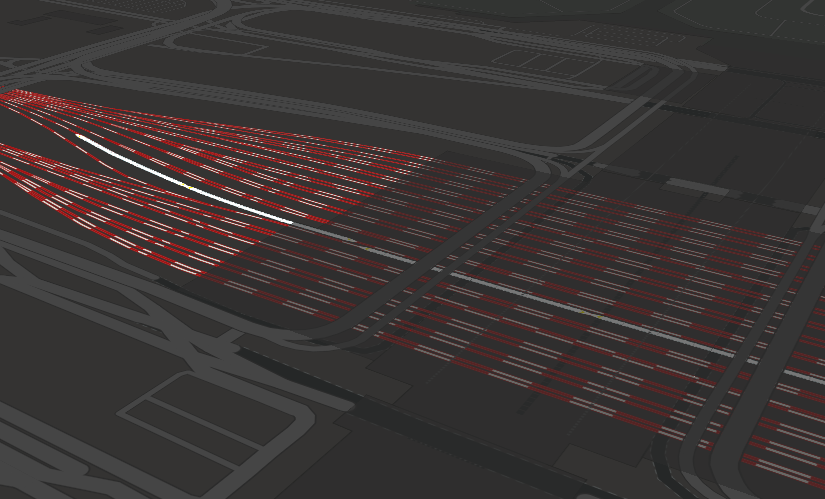# 虹桥火车站的火车运行可视化

## 可视化实现方法

### 列车模型

• 位置：经纬度表示的位置
• 旋转角度：车厢本身与正北之间的夹角
• 类型：车头，车身还是车尾
```static buildGeoJSON(coord, train_index, train_type, angle) {
var singe_type = "body";

if (train_type === "动车组" && (train_index===0 || train_index===8)) {
}
else if (train_type === "动车组" && (train_index===7 || train_index===15)) {
singe_type = "normal_tail";
}
else if (train_type === "高速铁路" && (train_index===0 || train_index===8)) {
}
else if (train_type === "高速铁路" && (train_index===7 || train_index===15)) {
singe_type = "high_tail";
}

return {
"type": "Feature",
"geometry": {
"type": "Point",
"coordinates": coord
},
"properties": {
"train_type": singe_type,
"icon_rotate": angle
}
};
}```

### 列车数据计算

```// 推算每节车厢的首尾位置的坐标
for(var i=this.route_path_coords.length-1; i > 0; i--) {
var coord1 = this.route_path_coords[i];
var coord2 = this.route_path_coords[i-1];
var tmp_distance = Train.calDistanceInM(coord1, coord1, coord2, coord2);
if(tmp_distance < passed_distance) {
passed_distance -= tmp_distance;
}
else {
result_coords.push(Train.getLocationByDistance(coord1, coord2, passed_distance/tmp_distance));
if (train_index > 0) {
train_index -= 1;
passed_distance += Train.single_length;
i += 1;
}
}

if (train_index === 0) {
break;
}
}

// 推算出每节车厢的坐标及旋转角度
for (var k=0; k<result_coords.length-1; k++) {
var coord1 = result_coords[k];
var coord2 = result_coords[k+1];
var center = [(coord1+coord2)/2, (coord1+coord2)/2];
var angle = Train.calAngle(coord1, coord1, coord2, coord2);

results.push(Train.buildGeoJSON(center, k, this.train_type, angle));
}```

## 代码获取

https://github.com/BranZhang/Hongqiao_railway_station

## 发布者### Zhang

hope you enjoy my articles.

## 《虹桥火车站的火车运行可视化》上有1条评论

1.匿名说道：

列车的实际到站信息在候车大厅内应该能获取到。